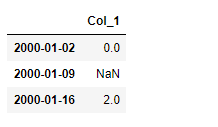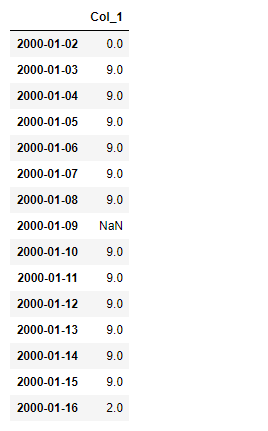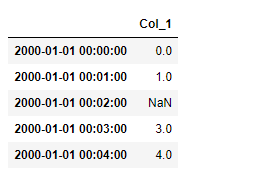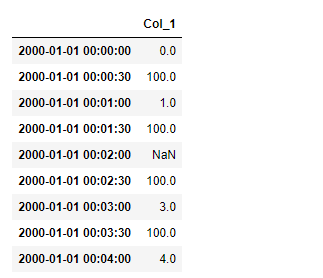# Python | Pandas dataframe.asfreq()

• Last Updated : 19 Nov, 2018

Python is a great language for doing data analysis, primarily because of the fantastic ecosystem of data-centric python packages. Pandas is one of those packages and makes importing and analyzing data much easier.

Pandas` dataframe.asfreq()` function is used to convert TimeSeries to specified frequency. This function Optionally provide filling method to pad/backfill missing values. It Returns the original data conformed to a new index with the specified frequency. resample is more appropriate if an operation, such as summarization, is necessary to represent the data at the new frequency.

Syntax : DataFrame.asfreq(freq, method=None, how=None, normalize=False, fill_value=None)

Parameters :
freq : DateOffset object, or string
method : Method to use for filling holes in reindexed Series
how : For PeriodIndex only, see PeriodIndex.asfreq
normalize : Whether to reset output index to midnight
fill_value : Value to use for missing values, applied during upsampling (note this does not fill NaNs that already were present).

Returns : converted : type of caller

Example #1: Unsample a time series data from weekly frequency to daily frequency

 `# importing pandas as pd``import` `pandas as pd`` ` `# Creating a date_time form index ``index_values ``=` `(pd.date_range(``'1/1/2000'``,``                   ``periods``=``3``,freq``=``'W'``))`` ` `# Creating a series using 'index_values'``# Notice, one of the series value is nan value``series ``=` `(pd.Series([``0.0``,``None``,``2.0``],``              ``index``=``index_values))`` ` `# Creating dataframe using the series``df``=``pd.DataFrame({``"Col_1"``:series})`` ` `# Print the Dataframe``df`Now unsample this weekly sampled data into daily sampled data.By default newly created bins will have nan value. So, use fill_value parameter to fill all newly created bins with a provided value.

 `# unsampling and providing a fill value = 9.0``df.asfreq(freq ``=``'D'``, fill_value ``=` `9.0``)`

Output :Note : This does not fill NaNs that already were present before sampling.

Example #2: Unsample a one minute timestamped data into 30s bins.First create a series with 5 one minute timestamps.

 `# importing pandas as pd``import` `pandas as pd`` ` `# Creating a date_time form index ``index_values ``=` `(pd.date_range(``'1/1/2000'``,``                     ``periods``=``5``,freq``=``'T'``))`` ` `# Creating a series using 'index_values'``# Notice, one of the series value is nan value``series ``=` `(pd.Series([``0.0``,``1.0``,``None``,``3.0``,``4.0``],``                      ``index``=``index_values))`` ` `# Creating dataframe using the series``df``=``pd.DataFrame({``"Col_1"``:series})`` ` `# Print the Dataframe``df`Now Unsampling into 30-second bins and providing a fill_value of 100.0

 `# unsampling and providing a fill value of 100.0``df.asfreq(freq ``=``'30S'``, fill_value ``=` `100.0``)`

Output :Note : Nan value present before unsampling will not be filled

My Personal Notes arrow_drop_up